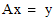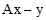# NMath User's Guide

21.3 Using Ordinary Least Squares Objects (.NET, C#, CSharp, VB, Visual Basic, F#)

Once a least squares object has been constructed from a matrix (Section 21.2), it may be used to solve least squares problems, if the factorization or decomposition was successful.

Testing for Goodness

Read-only properties are provided for determining whether the decomposition method was successful. The SVD least squares classes provide a Fail property that returns true if the SVD algorithm failed to converge.

Other methods are guaranteed to complete, but the resultant object may still be unusable for solving least squares problems, if for example the original matrix A was not of full rank. All least squares classes therefore provide an IsGood property that returns true if the method succeeded and the decomposition can be used to solve least squares problems.

Solving Least Squares Problems

All least squares classes provide a Solve() method that accepts a vector y, and computes the solution to the least squares problem. For example:

Code Example – C# least squares

```int rows = 6, cols = 3;
```
```var rng = new RandGenUniform( -2, 2 );

```

```DoubleMatrix A = GenerateData( rows, cols, rng );
```
```var lsq = new DoubleCholeskyLeastSq( A );

```

```var y = new DoubleVector( rows, rng );
```
```if ( lsq.IsGood )
```
```{
```
```  DoubleVector x = lsq.Solve( y );
```
```}
```

Code Example – VB least squares

```Dim Rows As Integer = 6
```
```Dim Cols As Integer = 3
```
```Dim RNG As New RandGenUniform(-2, 2)

```

```Dim A As DoubleMatrix = GenerateData(Rows, Cols, RNG)
```
```Dim LSQ As New DoubleCholeskyLeastSq(A)

```

```Dim Y As New DoubleVector(Rows, RNG)
```
```If LSQ.IsGood Then
```
```  Dim X As DoubleVector = LSQ.Solve(Y)
```
```End If
```

Method ResidualVector() returns the residual vector; ResidualNormSqr() computes the 2-norm squared of the residual vector. Finally, an existing least squares object can factor other matrices using the Factor() method.

Retrieving Information About the Original Matrix

Read-only properties are also provided for retrieving information about the original matrix A:

Rows gets the number of rows.

Cols gets the number of columns.

Rank (QR and SVD only) gets the numerical rank.

For example:

Code Example – C# least squares

```var A = new DoubleComplexMatrix(
```
```  "4x2[ (1,0) (0,0)  (0,0) (1,0)  (0,0) (0,0)  (0,0) (0,0) ]" );
```
```var lsq = new DoubleComplexQRLeastSq( A );
```
```int rank = lsq.Rank;
```

Code Example – VB least squares

```Dim A As New DoubleComplexMatrix(
```
```  "4x2[ (1,0) (0,0)  (0,0) (1,0)  (0,0) (0,0)  (0,0) (0,0) ]")
```
```Dim LSQ As New DoubleComplexQRLeastSq(A)
```
```Dim Rank As Integer = LSQ.Rank

```

Top

Top